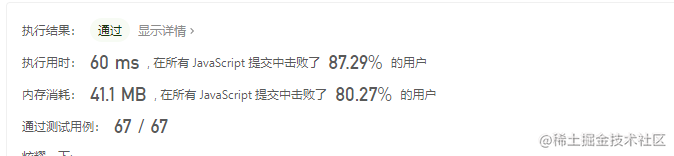# 1408. 数组中的字符串匹配

### 一、题目描述：

``````示例 1：

["hero","as"] 也是有效的答案。

### 二、思路分析：

#### 思路

1、通过 Join() 将数组链接成一个字符串 strs ；

2、如果 word[i] 是 另一个 word[j] 的子字符串，那么word[i]在 strs 出现的次数必定大于等于2，即 strs.indexOf(words[i])!=strs.lastIndexOf(words[i]);

3、循环遍历数组

### 三、AC 代码：

``````var stringMatching = function(words) {

let strs = words.join(",");

let res = []

for(let i=0; i< words.length; i++){

let first = strs.indexOf(words[i]);

let last = strs.lastIndexOf(words[i]);

if(first!=-1 &&last !=-1 && first!=last ){

res.push(words[i]);

}

}

return res;

}

### 四、总结：words 数组按照单字符串长度排序；

``````var stringMatching = function(words) {

words.sort((a,b) => a.length - b.length)

let set = new Set()

for(let i = 0;i < words.length - 1;i++) {

for(let j = i + 1;j < words.length;j++) {

if(words[j].indexOf(words[i]) > -1) {

}

}

}

return [...set]

};